# The farmer

The farmer calculated that the supply of fodder for his 20 cows was enough for 60 days. He decided to sell 2 cows and a third of the feed. How long will the feed for the rest of the peasant's herd last?

Correct result:

t =  44.444 d

#### Solution:

20•60 -20•60/3 = t•(20-2)

54t = 2400

t = 4009 ≈ 44.444444

Calculated by our simple equation calculator.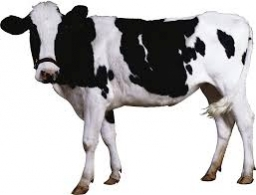Our examples were largely sent or created by pupils and students themselves. Therefore, we would be pleased if you could send us any errors you found, spelling mistakes, or rephasing the example. Thank you!

Leave us a comment of this math problem and its solution (i.e. if it is still somewhat unclear...):Be the first to comment!Tips to related online calculators
Do you have a linear equation or system of equations and looking for its solution? Or do you have quadratic equation?
Do you want to convert time units like minutes to seconds?
Most natural application of trigonometry and trigonometric functions is a calculation of the triangles. Common and less common calculations of different types of triangles offers our triangle calculator. Word trigonometry comes from Greek and literally means triangle calculation.

## Next similar math problems:

• A lot of hay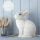Martin's grandfather weighed a lot of hay and calculated that for 15 rabbits it last in 100 days. How many days will this lot be enough for 25 rabbits?
• Worker's performance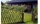15 workers paint 180 m fence in 3 days. In how many days will 9 workers paint a 360 m fence? We assume that each worker have the same, constant and unchangeable performance.
• Grandma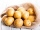6 kg of potatoes is enough for four at home for 15 days. For how many days will this amount be enough if a grandma will visit?
• The coal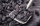The coal stock would be enough to heat a larger room for 12 weeks, a smaller one for 18 weeks. It was heated for four weeks in both rooms, then only in a smaller one. How long was the coal stock enough?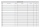Suzan reads a book. If she read half an hour a day, she would read it in nine days. How many minutes does he have to read a day if he wants to read it three days earlier?
• Bricklayers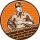The first bricklayer would build a wall in 3 days, the second in 4 days. The first bricklayer worked alone for a day, then the second bricklayer came to his aid and they finished the work together. How long did it take to build the wall?
• Working together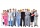Two people will do the work in 12 days. They worked together for 8 days. Then only one worked for 10 days. How many days would each of them do the work if he worked alone?
• Hectares of forest12 workers plant 24 ha of forest in 6 days. In how many days will 15 people and 12 people plant the same area?
• The orchard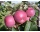4 temporary workers harvested the orchard in 9 days. How many temporary workers we need for six days?
• Five combers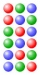Five combers harvest 12 rows of strawberries in 4 hours. How many rows of strawberries will two combers harvest in 10 hours?
• Cable carThe cable car will carry 1280 passengers in 40 minutes. How many people will be transported in 2.5 hours?
• The field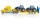3 tractors plow the field in 6 hours. However, after 2 hours, 1 tractor broke down. How long will the field be plowed?
• Work togetherTwo bricklayers plastering a wall. The first would plaster it in 8 hours, the second in 12 hours. How many hours will they be done with the work if they work together?
• Five tractorsFive tractors plow the fields in 4.8 hours. How long will it take to plow the field when, after an hour and a half of work, two tractor drivers have been called up for another task?
• Digging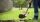The first worker would dig a trench in 6 hours. For the second, the same work would take 4 hours. How long did it take for the trench to be dug if they worked together?
• Seven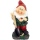Seven dwarfs will cut 420 stumps in 15 hours. After five hours, the two dwarves disappear discreetly. How many hours will the remaining dwarves complete the task?
• Baking muffins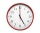Aunt Polly is baking muffins at a speed of 4 muffins per minute. Tom comes into the kitchen when Aunt Polly has 12 muffins, and begins to eat these muffins at a speed of 6 muffins per minute. When he finally leaves the kitchen, there are 4 muffins at the